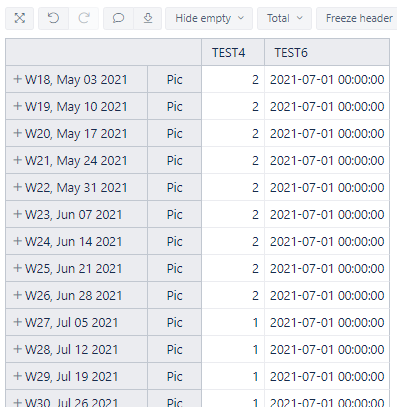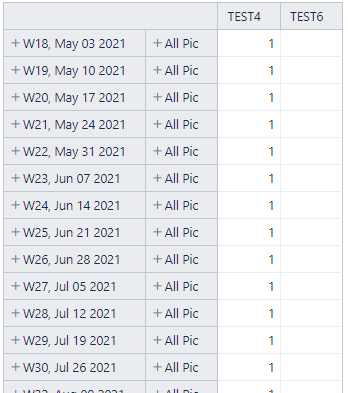# How can I get Date?

Hi
I want show [Time].[Start] in [Measures] Dimension.

[Time].[Start]
‘2021-06-31’

[Measures].[TEST4]
CASE
WHEN DateCompare([Time.Weekly].CurrentHierarchyMember.StartDate, DateParse([Time].[Start])) < 0
THEN 1
ELSE 2
END

So I get correct result like this.But I want not only “Pic” Project but also “Pic*” Projects.
So I create [Project].[All Pic].

[Project].[All Pic]
Aggregate(
Filter([Project].Members,
[Project].CurrentHierarchyMember.Name
MATCHES ‘^Pic.*’)
)

And then, EazyBI makes error like this.How can I get right result?

Hi @1115 ,

I recommend defining the date in Measures instead of a Time dimension calculated member. See the formula for a new calculated measure below:

`DateParse('2021-06-31')`

Use one of the “Date/Time” formatting options to display it as a date. See more details on formatting here - Calculated measures and members - eazyBI for Jira.

Then you can reference the calculated measure instead of the Time dimension calculated member in the formula TEST4, without DateParse().

What information do you want to display in the calculated measure “TEST6”?

Best,
Roberts // support@eazybi.com